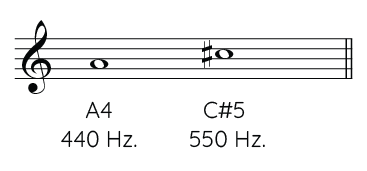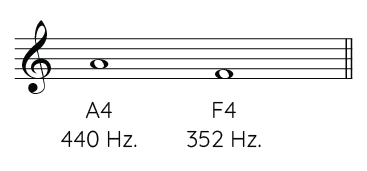#### The mathematical ratio of the intervals

The mathematical operation that we must use to determine the size of an interval is division. If we divide the frequencies, both thirds have the same size or mathematical ratio (1.25):

325 / 260 = 1.25

162.5 / 130 = 1.25

This number or mathematical ratio allows us to calculate major thirds based on any note. Multiplying we get an ascending major third and dividing descending. Starting from a 440 we obtain the upper C# and the lower F:

Note Frequency Ascending major third (C#5) Descending major third (F4)
A4 440 Hertz 440 * 1.25 = 550440 / 1.25 = 352